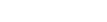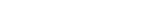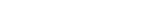Categories

How to calculate the R-squared in Python with and without sklearn

This tutorial is about calculating the R-squared in Python with and without the sklearn package.

For an exemplary calculation we are first defining two arrays. While the y_hat is the predicted y variable out of a linear regression, the y_true are the true y values.

import numpy as np

y_hat = np.array([2,3,5,7,2,3,8,5,3,1])
y_true = np.array([5,4,2,7,4,2,1,6,5,3])

Now we are calculating the R-squared out of those two variables.

The formulas for calculating the R-squared are:where SST is:and SSE is:To understand the SST and SSE consider the following image found on Wikipedia and created by Orzetto (Please see the credits and license below the image):